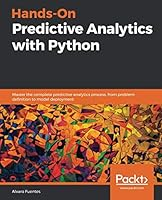# Hands-On Predictive Analytics with Python5 Reviews
2018-12-28
330 pages

## Book Description

Step-by-step guide to build high performing predictive applications

#### Key Features

• Use the data analytics ecosystem to implement end-to-end predictive analytics projects
• Explore advanced predictive modeling with an emphasis on theory with intuitive explanations
• Learn to deploy a predictive model's results as an interactive application

#### Book Description

Predictive analytics is an applied field that employs a variety of quantitative methods using data to make predictions. It involves much more than just throwing data onto a computer to build a model. This book provides practical coverage to help you understand the most important concepts of predictive analytics. Using practical, step-by-step examples, we build predictive analytics solutions while using cutting-edge Python tools and packages.

The book's step-by-step approach starts by defining the problem and moves on to identifying relevant data. We will also be performing data preparation, exploring and visualizing relationships, building models, tuning, evaluating, and deploying model.

Each stage has relevant practical examples and efficient Python . You will work with models such as KNN, Random Forests, and neural networks using the most important libraries in Python's data science stack: NumPy, Pandas, Matplotlib, Seaborn, Keras, Dash, and so on. In addition to hands-on examples, you will find intuitive explanations of the inner workings of the main techniques and algorithms used in predictive analytics.

By the end of this book, you will be all set to build high- predictive analytics solutions using Python programming.

#### What you will learn

• Get to grips with the main concepts and principles of predictive analytics
• Learn about the stages involved in producing complete predictive analytics solutions
• Understand how to define a problem, propose a solution, and prepare a dataset
• Use visualizations to explore relationships and gain insights into the dataset
• Learn to build regression and classification models using scikit-learn
• Use Keras to build powerful neural network models that produce accurate predictions
• Learn to serve a model's predictions as a web application

#### Who this book is for

This book is for data analysts, data scientists, data engineers, and Python developers who want to learn about predictive modeling and would like to implement predictive analytics solutions using Python's data stack. People from other backgrounds who would like to enter this exciting field will greatly benefit from reading this book. All you need is to be proficient in Python programming and have a basic understanding of statistics and -level algebra.

1. The Predictive Analytics Process
2. Problem Understanding and Data Preparation
3. Dataset Understanding - Exploratory
4. Predicting Numerical Values with Machine Learning
5. Predicting Categories with Machine Learning
6. Introducing Neural Nets for Predictive Analytics
7. Model Evaluation
8. Model Tuning and Improving Performance
9. Implementing a Model with Dash

## Book Details

• Title: Hands-On Predictive Analytics with Python
• Author:
• Length: 330 pages
• Edition: 1
• Language: English
• Publisher:
• Publication Date: 2018-12-28
• ISBN-10: 178913871X
• ISBN-13: 9781789138719